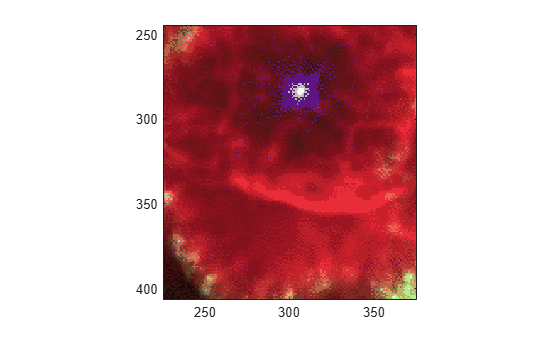Documentation

### This is machine translation

Mouseover text to see original. Click the button below to return to the English version of the page.

To view all translated materials including this page, select Country from the country navigator on the bottom of this page.

# rgb2ind

Convert RGB image to indexed image

## Syntax

```[X,map] = rgb2ind(RGB,n) X = rgb2ind(RGB, map) [X,map] = rgb2ind(RGB, tol) [___] = rgb2ind(___,dither_option) ```

## Description

`[X,map] = rgb2ind(RGB,n)` converts the RGB image to an indexed image `X` using minimum variance quantization and dithering. `map` contains at most `n` colors. `n` must be less than or equal to 65,536.

`X = rgb2ind(RGB, map)` converts the RGB image to an indexed image `X` with colormap `map` using the inverse colormap algorithm and dithering. `size(map,1)` must be less than or equal to 65,536.

`[X,map] = rgb2ind(RGB, tol)` converts the RGB image to an indexed image `X` using uniform quantization and dithering. `map` contains at most `(floor(1/tol)+1)^3` colors. `tol` must be between 0.0 and 1.0.

`[___] = rgb2ind(___,dither_option)` enables or disables dithering. `dither_option` can be one of these values.

 `'dither'` (default) Dithers, if necessary, to achieve better color resolution at the expense of spatial resolution `'nodither'` Maps each color in the original image to the closest color in the new map. No dithering is performed.

### Note

The values in the resultant image `X` are indexes into the colormap `map` and should not be used in mathematical processing, such as filtering operations.

## Class Support

The input image can be of class `uint8`, `uint16`, `single`, or `double`. If the length of `map` is less than or equal to 256, the output image is of class `uint8`. Otherwise, the output image is of class `uint16`.

The value `0` in the output array `X` corresponds to the first color in the colormap.

## Examples

collapse all

Read and display a truecolor `uint8` JPEG image of a nebula.

```RGB = imread('ngc6543a.jpg'); figure imagesc(RGB) axis image zoom(4)```Convert RGB to an indexed image with 32 colors.

```[IND,map] = rgb2ind(RGB,32); figure imagesc(IND) colormap(map) axis image zoom(4)```## Tips

• If you specify `tol`, `rgb2ind` uses uniform quantization to convert the image. This method involves cutting the RGB color cube into smaller cubes of length `tol`.

• If you specify `n`, `rgb2ind` uses minimum variance quantization. This method involves cutting the RGB color cube into smaller boxes (not necessarily cubes) of different sizes, depending on how the colors are distributed in the image. If the input image actually uses fewer colors than the number you specify, the output colormap is also smaller.

• If you specify `map`, `rgb2ind` uses colormap mapping, which involves finding the colors in `map` that best match the colors in the RGB image.

## Algorithms

• Uniform Quantization — Uniform quantization cuts the RGB color cube into smaller cubes of length `tol`. For example, if you specify a `tol` of 0.1, the edges of the cubes are one-tenth the length of the RGB cube. The total number of small cubes is:

```n = (floor(1/tol)+1)^3 ```

Each cube represents a single color in the output image. Therefore, the maximum length of the colormap is `n`. `rgb2ind` removes any colors that don’t appear in the input image, so the actual colormap can be much smaller than `n`.

• Minimum Variance Quantization — Minimum variance quantization cuts the RGB color cube into smaller boxes (not necessarily cubes) of different sizes, depending on how the colors are distributed in the image. If the input image actually uses fewer colors than the number specified, the output colormap is also smaller.

• Inverse Colormap — The inverse colormap algorithm quantizes the specified colormap into 32 distinct levels per color component. Then, for each pixel in the input image, the closest color in the quantized colormap is found.

 Spencer W. Thomas, "Efficient Inverse Color Map Computation", Graphics Gems II, (ed. James Arvo), Academic Press: Boston. 1991. (includes source code)